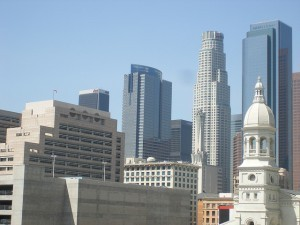# Biggest Cities in California

The following list of the biggest cities in California is based on population. Keep in mind that these figures are estimates only.

### The Top Ten

The biggest city is Los Angeles with a population of 4,094,764. At number 2 is San Diego. Its population has been estimated at 1,376,173. San Jose occupies the third spot with a population of 1,023,083.

At number four is San Francisco. The city has a population of 856,095. The fifth most populated city in California is Fresno. It has an estimated population of 505,479. Long Beach is at number six. It has 501,709 inhabitants.

Sacramento is number seven. Its population has been estimated to be 500,189. At number eight is Oakland. Oakland’s population has been estimated to be 430,666. Santa Ana is at number nine with a population of 357,754. The tenth spot is occupied by Anaheim. Anaheim’s population has been put at 353,643.

### The Biggest Cities in California: 11 to 20

Bakersfield is at number 11. The population has been pegged at 338,952. Riverside is at number 12 with 304,051. At number 13 is Stockton. The population is 292,133. Chula Vista is at number 14. The population estimate is 237,595.Fremont is at number 15. The population figure has been put at 218,128. At number 16 is Irvine. The population is 217,686. The 17th most populated city is Modesto. The population is 211,536.

Glendale is at number 18 with 207,902. San Bernardino is at number 19. The population is 204,800. The 20th slot is occupied by Huntington Beach. The population is 203,484.

### Number 21 to 35

Oxnard is at number 21. The population is 200,004. Fontana is at number 22. The population is 190,356 and Moreno Valley is at number 23. Its population is 188,537. Oceanside is at number 24. Its population is 183,095. Rancho Cucamonga is at number 25. The population is 178,904.

At number 26 is Santa Clarita with 177,641. Garden Grove is at the 27th slot. It has a population of 175,618. Ontario is at number 28 with 174,536. At number 29 is Pomona with 163,683. At number 30 is Santa Rosa. The population is 163,436. At number 31 is Salinas. The population is 153,948.

At number 32 is Hayward is 153,104. At number 33 in the list of the biggest cities in California is Palmdale with 152,622. The 34th slot belongs to Pasadena with 151,576. At number 35 is Corona with 150,416.